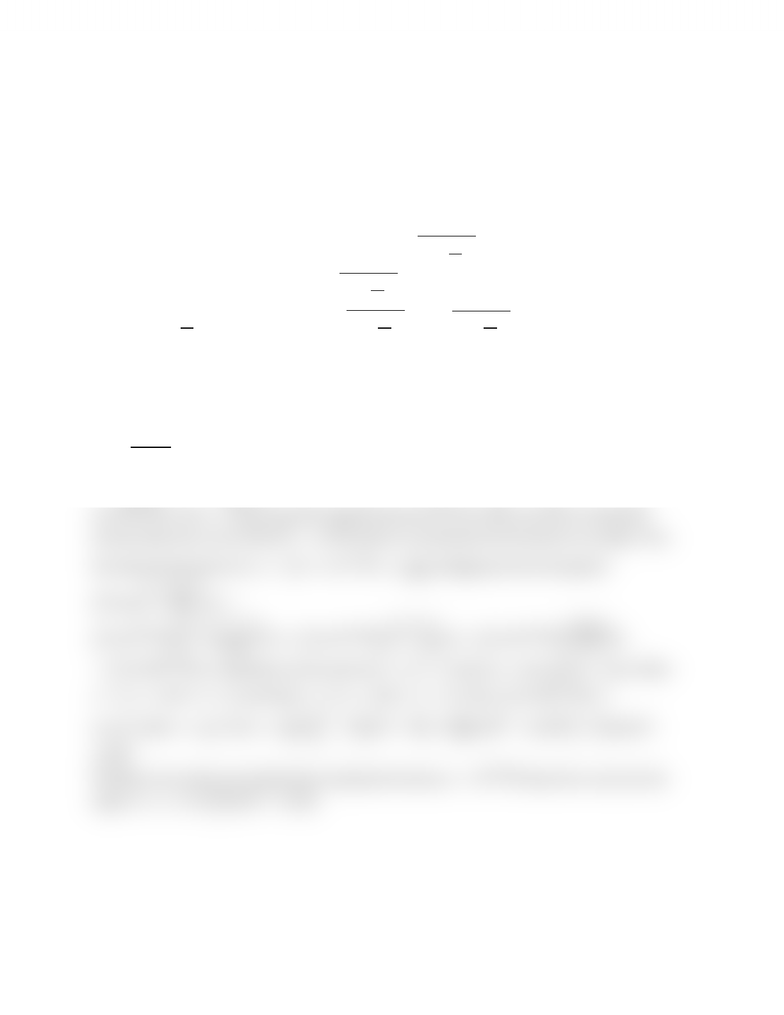Class Notes (1,100,000)
CA (630,000)
UTSG (50,000)
MAT (4,000)
MAT136H1 (900)
all (200)
Lecture

8.2 Surface Area of Revolution Question #1 (Easy)

Department
Mathematics
Course Code
MAT136H1
Professor
all

This preview shows half of the first page. to view the full 1 pages of the document.8.2 Challenging Integral Applications
Surface Area of Revolution
Question #1 (Easy): Finding the Surface Area From Rotating the Function About the X-Axis
Strategy
When the function is rotated about the -axis, then the radius extends vertically up. When the function
is expressed as  , then use the formula 

. But if the function is given in the
form of  , then use  

. The part that comes from arc length can be
evaluated with 
 because based on  
 
. So other than
rearrangement of the function is needed to express the vertically stretching radius, but as for the ds
portion, the derivative can be taken as it is.
Sample Question
Find the exact surface area obtained by rotating the given function about the -axis.
  ,    
Solution
From    comes the integral expression for the surface area from rotating the
function about the x-axis: 
. Notice it incorporates the formula for arc length. Thus,
first take the derivative of y:

. Plugging into the SA equation:







 

. substitution can be used. let  , then   and 
. When
 ,    , and when  ,    . Then: 





 





Therefore, the surface area obtained by rotating the function   about the x-axis over the
region    is 

You're Reading a Preview

Unlock to view full version